# 1.4 Inverse functions  (Page 5/11)

 Page 5 / 11

## The maximum value of a function

In many areas of science, engineering, and mathematics, it is useful to know the maximum value a function can obtain, even if we don’t know its exact value at a given instant. For instance, if we have a function describing the strength of a roof beam, we would want to know the maximum weight the beam can support without breaking. If we have a function that describes the speed of a train, we would want to know its maximum speed before it jumps off the rails. Safe design often depends on knowing maximum values.

This project describes a simple example of a function with a maximum value that depends on two equation coefficients. We will see that maximum values can depend on several factors other than the independent variable x .

1. Consider the graph in [link] of the function $y=\text{sin}\phantom{\rule{0.1em}{0ex}}x+\text{cos}\phantom{\rule{0.1em}{0ex}}x.$ Describe its overall shape. Is it periodic? How do you know?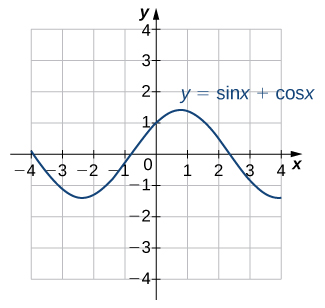The graph of y = sin x + cos x .
Using a graphing calculator or other graphing device, estimate the $x$ - and $y$ -values of the maximum point for the graph (the first such point where x >0). It may be helpful to express the $x$ -value as a multiple of π.
2. Now consider other graphs of the form $y=A\phantom{\rule{0.1em}{0ex}}\text{sin}\phantom{\rule{0.1em}{0ex}}x+B\phantom{\rule{0.1em}{0ex}}\text{cos}\phantom{\rule{0.1em}{0ex}}x$ for various values of A and B . Sketch the graph when A = 2 and B = 1, and find the $x$ - and y -values for the maximum point. (Remember to express the x -value as a multiple of π, if possible.) Has it moved?
3. Repeat for A = 1, B = 2. Is there any relationship to what you found in part (2)?
4. Complete the following table, adding a few choices of your own for A and B :
A B x y A B x y
0 1 $\sqrt{3}$ 1
1 0 1 $\sqrt{3}$
1 1 12 5
1 2 5 12
2 1
2 2
3 4
4 3
5. Try to figure out the formula for the y -values.
6. The formula for the $x$ -values is a little harder. The most helpful points from the table are $\left(1,1\right),\left(1,\sqrt{3}\right),\left(\sqrt{3},1\right).$ ( Hint : Consider inverse trigonometric functions.)
7. If you found formulas for parts (5) and (6), show that they work together. That is, substitute the $x$ -value formula you found into $y=A\phantom{\rule{0.1em}{0ex}}\text{sin}\phantom{\rule{0.1em}{0ex}}x+B\phantom{\rule{0.1em}{0ex}}\text{cos}\phantom{\rule{0.1em}{0ex}}x$ and simplify it to arrive at the $y$ -value formula you found.

## Key concepts

• For a function to have an inverse, the function must be one-to-one. Given the graph of a function, we can determine whether the function is one-to-one by using the horizontal line test.
• If a function is not one-to-one, we can restrict the domain to a smaller domain where the function is one-to-one and then define the inverse of the function on the smaller domain.
• For a function $f$ and its inverse ${f}^{-1},f\left({f}^{-1}\left(x\right)\right)=x$ for all $x$ in the domain of ${f}^{-1}$ and ${f}^{-1}\left(f\left(x\right)\right)=x$ for all $x$ in the domain of $f.$
• Since the trigonometric functions are periodic, we need to restrict their domains to define the inverse trigonometric functions.
• The graph of a function $f$ and its inverse ${f}^{-1}$ are symmetric about the line $y=x.$

## Key equations

• Inverse functions
${f}^{-1}\left(f\left(x\right)\right)=x\phantom{\rule{0.2em}{0ex}}\text{for all}\phantom{\rule{0.2em}{0ex}}x\phantom{\rule{0.2em}{0ex}}\text{in}\phantom{\rule{0.2em}{0ex}}D,\text{and}\phantom{\rule{0.2em}{0ex}}f\left({f}^{-1}\left(y\right)\right)=y\phantom{\rule{0.2em}{0ex}}\text{for all}\phantom{\rule{0.2em}{0ex}}y\phantom{\rule{0.2em}{0ex}}\text{in}\phantom{\rule{0.2em}{0ex}}R.$

For the following exercises, use the horizontal line test to determine whether each of the given graphs is one-to-one.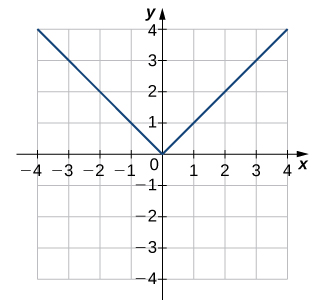Not one-to-one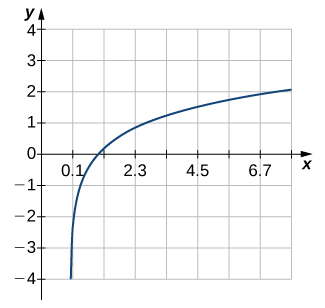Not one-to-one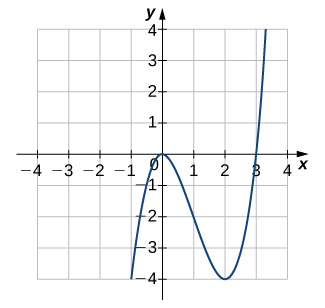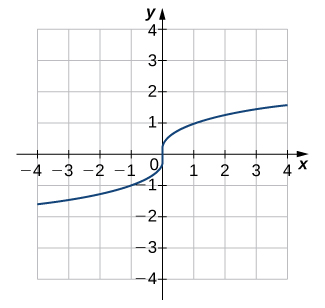One-to-one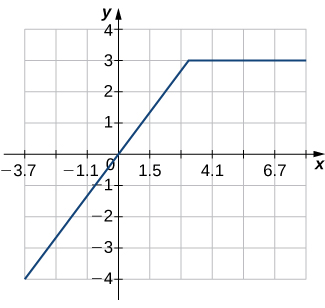For the following exercises, a. find the inverse function, and b. find the domain and range of the inverse function.

$f\left(x\right)={x}^{2}-4,x\ge 0$

a. ${f}^{-1}\left(x\right)=\sqrt{x+4}$ b. Domain $\text{:}\phantom{\rule{0.2em}{0ex}}x\ge -4,\text{range}\text{:}\phantom{\rule{0.2em}{0ex}}y\ge 0$

f(x) = x-2 g(x) = 3x + 5 fog(x)? f(x)/g(x)
fog(x)= f(g(x)) = x-2 = 3x+5-2 = 3x+3 f(x)/g(x)= x-2/3x+5
diron
pweding paturo nsa calculus?
jimmy
how to use fundamental theorem to solve exponential
find the bounded area of the parabola y^2=4x and y=16x
what is absolute value means?
Chicken nuggets
Hugh
🐔
MM
🐔🦃 nuggets
MM
(mathematics) For a complex number a+bi, the principal square root of the sum of the squares of its real and imaginary parts, √a2+b2 . Denoted by | |. The absolute value |x| of a real number x is √x2 , which is equal to x if x is non-negative, and −x if x is negative.
Ismael
find integration of loge x
find the volume of a solid about the y-axis, x=0, x=1, y=0, y=7+x^3
how does this work
Can calculus give the answers as same as other methods give in basic classes while solving the numericals?
log tan (x/4+x/2)
Rohan
Rohan
y=(x^2 + 3x).(eipix)
Claudia
Ismael
A Function F(X)=Sinx+cosx is odd or even?
neither
David
Neither
Lovuyiso
f(x)=1/1+x^2 |=[-3,1]
apa itu?
fauzi
determine the area of the region enclosed by x²+y=1,2x-y+4=0
Hi
MP
Hi too
Vic
hello please anyone with calculus PDF should share
Which kind of pdf do you want bro?
Aftab
hi
Abdul
can I get calculus in pdf
Abdul
explain for me
Usman
okay I have such documents
Fitzgerald
Hamza
How to use it to slove fraction
Hello please can someone tell me the meaning of this group all about, yes I know is calculus group but yet nothing is showing up
Shodipo
You have downloaded the aplication Calculus Volume 1, tackling about lessons for (mostly) college freshmen, Calculus 1: Differential, and this group I think aims to let concerns and questions from students who want to clarify something about the subject. Well, this is what I guess so.
Jean
Im not in college but this will still help
nothing
how en where can u apply it
Migos
how can we scatch a parabola graph
Ok
Endalkachew
how can I solve differentiation?
with the help of different formulas and Rules. we use formulas according to given condition or according to questions
CALCULUS
For example any questions...
CALCULUS
v=(x,y) وu=(x,y ) ∂u/∂x* ∂x/∂u +∂v/∂x*∂x/∂v=1
log tan (x/4+x/2)
Rohan
what is the procedures in solving number 1?

#### Get Jobilize Job Search Mobile App in your pocket Now!By OpenStaxBy John GabrieliBy OpenStaxBy OpenStaxBy OpenStaxBy JavaChamp TeamBy P. Wynn NormanBy Sarah WarrenBy Steve GibbsBy Stephen Voron